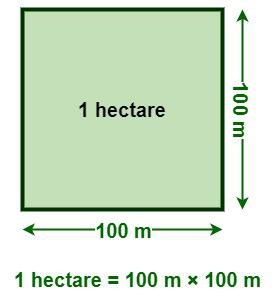Open in App
Not now

# What is Hectare?

• Last Updated : 17 Nov, 2022

A hectare is a unit of measurement for an area. The space occupied by a flat shape or an object’s surface is referred to as its area. Hectare is used to measure the area of a square, the area of a triangle, and other objects. The area of a two-dimensional shape varies from one shape to another, as we have different shapes like circles, squares, rectangles, etc. A square meter is the SI unit of the area of a geometric shape, where one square meter is the area of a square with measurements of 1 meter long and 1 meter wide. In this article, we will discuss the definition of a hectare and its conversion to other units.

## Hectare Definition

A hectare is a non-SI unit of area measurement accepted for use with the SI that is expressed as “ha.” It is widely used all around the world to measure large areas of land. One hectare is the area of a square with measurements of 100 meters long and 100 meters wide. The word “Hectare” was first used in French, from the Latin ārea. One square kilometer equals 100 hectares. The value of one hectare is exactly equal to 100 ares, 10,000 square meters, 0.01 square kilometers, and approximately equal to 2.4711 acres, 247.105 cents, 11,960 square yards, and 107639.104 square feet.## Conversion of Hectare

The following table is used to convert Hectare to other units.

## Hectares to Square Meters

The value of 1 hectare is exactly equal to 10000 square meters. So, to convert hectares to square meters, we have to multiply the given value by 10000 square meters.

1 Hectare = 10000 square meters

### How to convert Hectares to Square Meters?

Follow the steps below to convert Hectares to Square Meters.

Example: Convert 21 hectares to square meters.

Step 1: Multiply 21 by 10000 square meters, i.e., 21 hectares = 21 × 10000 square meters

Step 2: Calculate the value.

21 hectares = 2,10,000 square meters

Thus, 21 hectares is equal to 2,10,000 square meters.

## Hectares to Ares

The value of 1 hectare is exactly equal to 100 ares. So, to convert hectares to ares, we have to multiply the given value by 100 ares.

1 Hectare = 100 ares

### How to convert Hectares to Ares?

Follow the steps given below to convert Hectares to Ares.

Example: Convert 21 Hectares to Ares.

Step 1: Multiply 21 by 100 ares, i.e., 21 hectares = 21 × 100 ares

Step 2: Calculate the value.

21 hectares = 2100 ares

Thus, 21 hectares is equal to 2100 ares.

## Hectares to Acres

The value of 1 hectare is approximately equal to 2.471 acres. So, to convert hectares to acres, we have to multiply the given value by 2.471 acres.

1 hectare = 2.471 acres

### How to convert Hectares to Acres?

Follow the steps given below to convert Hectares to Acres.

Example: Convert 21 hectares to acres.

Step 1: Multiply 21 by 2.471 acres, i.e., 21 hectares = 21 × 2.471 acres

Step 2: Calculate the value.

21 hectares = 51.891 acres

Thus, 21 hectares is approximately equal to 51.891 acres.

## Hectares to Cents

1 Hectare = 247.10514 Cents

### How to convert Hectares to Cents?

Follow the steps given below to convert Hectares to Cents.

Example: Convert 21 hectares to Cents.

Step 1: Multiply 21 by 247.105 Cents, i.e., 21 hectares = 21 × 247.10514 Cents

Step 2: Calculate the value.

21 hectares = 5,189.20794 cents

Thus, 21 hectares is approximately equal to 5,189.205 cents.

## Solved Problems on Conversion of Hectares

Problem 1: Convert 15 hectares into acres.

Solution:

We know that,

1 hectare = 2.471 acres

Hence,

15 hectares = 15 × 2.471 acres

15 hectares = 37.065 acres

Therefore, 15 hectares is approximately equal to 37.065 acres.

Problem 2: Convert 67 hectares into cents.

Solution:

We know that,

1 Hectare = 247.105 cents

Hence,

67 hectares = 67 × 247.105 cents

67 hectares = 16,556.035 cents

Therefore, 67 hectares is approximately equal to 16,556.035 cents.

Problem 3: Convert 34 acres into hectares.

Solution:

We know that,

1 acre = 0.40468564 hectares

Hence,

34 acres = 34 × 0.40468564 hectares

34 acres = 13.75931176 hectares

34 acres = 13.76 hectares

Therefore, 0.153 acres is approximately equal to 13.76 hectares.

Problem 4: Convert 1.25 square kilometers into hectares.

Solution:

We know that,

1 square kilometer = 100 hectares

Hence,

1.25 square kilometers = 1.25 × 100 hectares

1.25 square kilometers = 125 hectares

Therefore, 1.25 square kilometers is equal to 125 hectares.

Problem 5: Convert 2.7 hectares into ares.

Solution:

We know that,

1 hectare = 100 ares

Hence,

2.7 hectares = 2.7 × 100 ares

2.7 hectares = 270 ares

Therefore, 2.7 hectares is approximately equal to 270 ares.

## FAQs on Hectare

Question 1: What is a hectare?

A hectare is a non-SI unit of area measurement accepted for use with the SI that is expressed as “ha.” It is widely used all around the world to measure large areas of land. One hectare is the area of a square with measurements of 100 meters long and 100 meters wide.

1 Hectare = 100m × 100m

Question 2: How can we convert square kilometers to hectares?

One square kilometer equals 100 hectares. So, the relationship between square kilometers and hectares is given as follows:

1 square kilometer = 100 hectares

Question 3: How can we convert hectares to acres?

The value of 1 hectare is approximately equal to 2.471 acres. So, to convert hectares to acres, we have to multiply the given value by 2.471 acres.

1 hectare = 2.471 acres

Question 4: How can we convert hectares to square meters?